## Rotational Symmetry

If the potential only depends on the distance between two particles,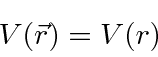the Hamiltonian has Rotational Symmetry. This is true for the coulomb (and gravitational) potential as well as many others. We know from classical mechanics that these are important problems.

If a the Hamiltonian has rotational symmetry, we can show that the Angular Momentum operators commute with the Hamiltonian.We therefore expect each component of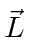to be conserved.

We will not be able to label our states with the quantum numbers for the three components of angular momentum. Recall that we are looking for a set of mutually commuting operators to label our energy eigenstates. We actually want two operators plus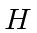to give us three quantum numbers for states in three dimensions.

The components of angular momentum do not commute with each otherbut the square of the angular momentum commutes with any of the componentsThese commutators lead us to choose the mutually commuting set of operators to be,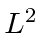, and. We could have chosen any component, however, it is most convenient to choosegiven the standard definition of spherical coordinates.

The Schrödinger equation now can be rewritten with only radial derivatives and.This leads to a great simplification of the 3D problem.

It is possible to separate the Schrödinger equation sinceandappear separately. Write the solution as a product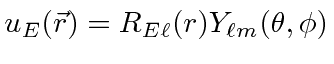wherelabels the eigenvalue of theoperator andlabels the eigenvalue of theoperator. Sincedoes not appear in the Schrödinger equation, we only label the radial solutions with the energy and the eigenvalues of.

We get the three equations.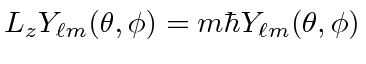By assuming the eigenvalues ofhave the form, we have anticipated the solution but not constrained it, since the units of angular momentum are those of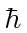and since we expectto have positive eigenvalues.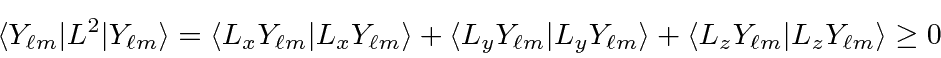The assumption that the eigenvalues ofare some (dimensionless) number timesdoes not constrain our solutions at all.

We will use the algebra of the angular momentum operators to help us solve the angular part of the problem in general.

For any given problem with rotational symmetry, we will need to solve a particular differential equation in one variable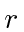. This radial equation can be simplified a bit.We have grouped the term due to angular momentum with the potential. It is often called a pseudo-potential. For, it is like a repulsive potential.

Jim Branson 2013-04-22